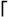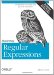# Section 7.4. The qr Operator and Regex Objects

### 7.4. The qr/‹/ Operator and Regex Objects

Introduced briefly in Chapter 2 and Chapter 6 (˜76; 277), qr/‹/ is a unary operator that takes a regex operand and returns a regex object . The returned object can then be used as a regex operand of a later match, substitution, or split , or can be used as a sub-part of a larger regex.

Regex objects are used primarily to encapsulate a regex into a unit that can be used to build larger expressions, and for efficiency (to gain control over exactly when a regex is compiled, discussed later).

As described on page 291, you can pick your own delimiters, such as qr{‹} or qr!‹! . It supports the core modifiers /i, /x, /s, /m , and /o .

#### 7.4.1. Building and Using Regex Objects

Consider the following, with expressions adapted from Chapter 2 (˜76):

` my  \$HostnameRegex  =  qr/  [-a-z0-9]+(? :\.[-a-z0-9]+)+\.(?: comeduinfo)/  i  ;     my \$HttpUrl     =  qr{  http://  \$HostnameRegex  \b #  Hostname  (?:              / [ - a-z0-9R:\@&? = +,.!/~*'%\$]* #  Optional path  (?<![.,?!]) #  Not allowed to end with [.,?!]  )?  }ix;  `

The first line encapsulates our simplistic hostname-matching regex into a regular-expression object, and saves it to the variable \$HostnameRegex . The next lines then use that in building a regex object to match an HTTP URL, saved to the variable \$HttpUrl . Once constructed , they can be used in a variety of ways, such as

` if (\$text =~ \$HttpUrl) {        print "There is a URL\n";     } `

to merely inspect, or perhaps

` while (\$text =~  m  /(\$HttpUrl)/  g  ) {        print "Found URL: \n";     } `

to find and display all HTTP URLs.

Now, consider changing the definition of \$HostnameRegex to this, derived from Chapter 5 (˜205):

` my \$HostnameRegex = qr{        # One or more dot-separated parts        (?: [a-z0-9]\.  [a-z0-9][-a-z0-9]{0,61}[a-z0-9]\.) *        # Followed by the final suffix part        (?: comedugovintmilnetorgbizinfoaero[a-z][a-z])     }xi; `

This is intended to be used in the same way as our previous version (for example, it doesn't have a leading^and trailing\$, and has no capturing parentheses), so were free to use it as a drop-in replacement. Doing so gives us a stronger \$HttpUrl .

##### 7.4.1.1. Match modes (or lack thereof) are very sticky

qr/‹/ supports the core modifiers described on page 292. Once a regex object is built, the match modes of the regex it represents can't be changed, even if that regex object is used inside a subsequent m/‹/ that has its own modifiers. For example, the following does not work:

` my \$WordRegex = qr/\b \w+ \b/; #  Oops, missing the /x modifier!if (\$text =~ m/^(\$WordRegex)/  x  ) {         print "found word at start of text: \n";     } `

The /x modifiers are used here ostensibly to modify how \$WordRegex is applied , but this does not work because the modifiers (or lack thereof) are locked in by the qr/‹/ when \$WordRegex is created . So, the appropriate modifiers must be used at that time.

Here's a working version of the previous example:

` my \$WordRegex = qr/\b \  w+  \b/  x  ; #  This works!if (\$text =~ m/^(\$WordRegex)/) {         print "found word at start of text: \n";     } `

Now, contrast the original snippet with the following:

` my \$WordRegex = '\b \w+ \b'; #  Normal string assignmentif (\$text =~ m/^(\$WordRegex)/  x  ) {         print "found word at start of text: \n";     } `

Unlike the original, this one works even though no modifiers are associated with \$WordRegex when it is created. That's because in this case, \$WordRegex is a normal variable holding a simple string that is interpolated into the m/‹/ regex literal. Building up a regex in a string is much less convenient than using regex objects, for a variety of reasons, including the problem in this case of having to remember that this \$WordRegex must be applied with /x to be useful.

Actually, you can solve that problem even when using strings by putting the regex into a mode-modified span (˜135) when creating the string:

` my \$WordRegex = '  (?x:  \b \w+ \b)'; #  Normal string assignmentif (\$text =~ m/^(\$WordRegex)/) {         print "found word at start of text: \n";     } `

In this case, after the m/‹/ regex literal interpolates the string, the regex engine is presented with^((?x: \b \w+ \b ) ), which works the way we want.

In fact, this is what logically happens when a regex object is created, except that a regex object always explicitly defines the "on" or "off" for each of the /i, /x, /m , and /s modes. Using qr/\b \w+ \b/x creates(?x-ism: \b \w+ \b ). Notice how the mode-modified span,(? x -ism:‹), has /x turned on, while /i, /s , and /m are turned off. Thus, qr/‹/ always "locks in each mode, whether given a modifier or not.

#### 7.4.2. Viewing Regex Objects

The previous paragraph talks about how regex objects logically wrap their regular expression with mode-modified spans like(?x-ism:‹). You can actually see this for yourself, because if you use a regex object where Perl expects a string, Perl kindly gives a textual representation of the regex it represents. For example:

` %  perl -e 'print qr/\b \w+ \b/x, "\n"'  (?x-ism:\b \w+ \b) `

Here's what we get when we print the \$HttpUrl from page 304:

` (?ix-sm:        http:// (?ix-sm:        # One or more dot-separated parts        (?: [a-z0-9]\.  [a-z0-9][-a-z0-9]{0,61}[a-z0-9]\.) *        # Followed by the final suffix part        (?: comedugovintmilnetorgbizinfoaero[a-z][a-z])) \b # hostname         (?:             / [ - a-z0-9R:\@&? =+,.!/~*'%\$]* # Optional path                   (?<![.,?!]) # Not allowed to end with [.,?!])?) `

The ability to turn a regex object into a string is very useful for debugging.

#### 7.4.3. Using Regex Objects for Efficiency

One of the main reasons to use regex objects is to gain control, for efficiency reasons, of exactly when Perl compiles a regex to an internal form. The general issue of regex compilation was discussed briefly in Chapter 6, but the more complex Perl- related issues, including regex objects, are discussed in "Regex Compilation, the /o Modifier, qr/‹/ , and Efficiency" (˜348).Mastering Regular Expressions
ISBN: 0596528124
EAN: 2147483647
Year: 2004
Pages: 113

Similar book on Amazon